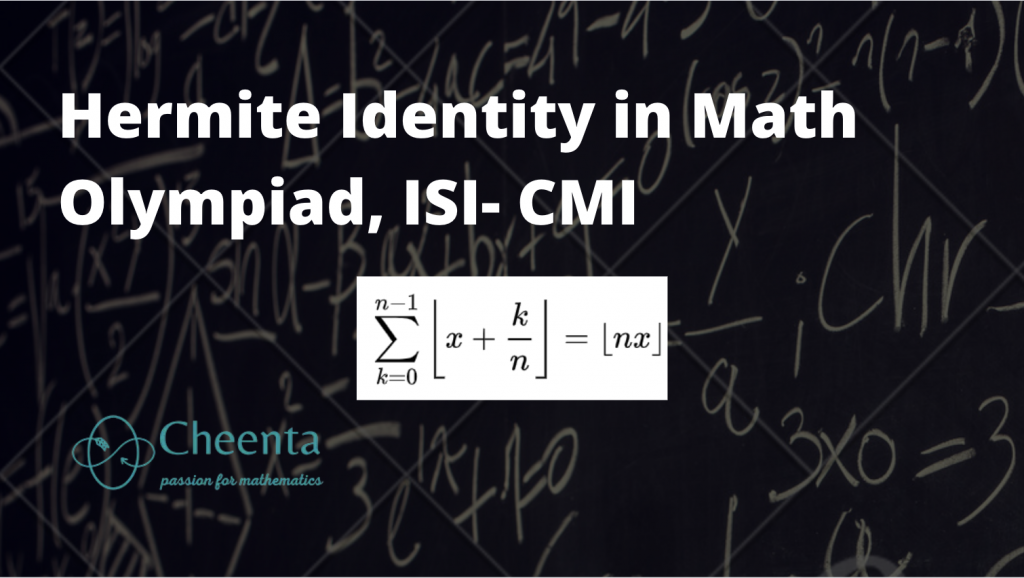How Cheenta works to ensure student success?
Explore the Back-Story

# Hermite Identity in Math Olympiad, ISI CMI EntranceIn mathematics, periodic functions play a very important role. Examples include $f(x) = \sin (x)$, $f(x) = \{x \}$ and so on. We discuss an interesting property of greatest integer function using the technique periodicity.

In mathematics, periodic functions play a very important role. Examples include $f(x) = \sin (x)$, $f(x) = \{x \}$ and so on. We discuss an interesting property of greatest integer function using the technique periodicity.

This site uses Akismet to reduce spam. Learn how your comment data is processed.

### Knowledge Partner